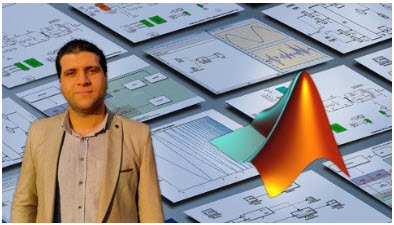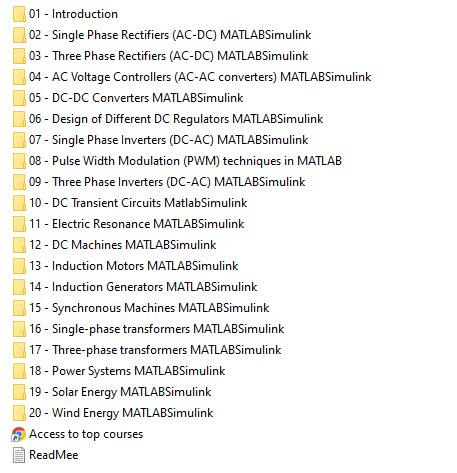Tutorials

# Ultimate MATLAB-Simulink for Electrical EngineeriningGenre: eLearning | MP4 | Video: h264, 1280×720 | Audio: AAC, 44.1 KHz
Language: English | Size: 10.2 GB | Duration: 124 lectures • 27h 19m

What you’ll learn
Learn MATLAB simulations related to electrical enegineering topics
Complete MATLAB simulations of power electronics
Simulations of 1-phase rectifiers (controlled, uncontrolled, HWR and FWR)
How to simulate 3-phase rectifiers in MATLAB-Simulink
Complete Simulations of AC voltage controllers ( 1-ph & 3-ph)
How to apply closed loop control of AC voltage controllers in MATLAB-Simulink
Complete MATLAB simulations of DC-DC converters
How to apply PID controller to DC-DC converters in MATLAB/Simulink
How to apply Hystereysis controller to DC-DC converters in MATLAB/Simulink
How to design different DC Regulators in MATLAB/Simulink
How to simulate 1-ph inverter circuits (half bridge and full bridge)
How to apply pulse width modulation (PWM) techniques in MATLAB/Simulink
How to simulate 3-ph inverter circuits
Simulations of R-L , R-C , R-L-C circuits in MATLAB/Simulink
Applying forced and free response in transient circuits in Simulink
How to simulate the electric resonance in MATLAB/Simulink
Complete MATLAB Simulations of DC motors (shunt, series and separately excited)
How to apply speed control of DC motors in MATLAB/Simulink
How to start DC motors using starters in MATLAB/Simulink
Complete simulations of Induction motors
Learn speed control and starting of induction motors in MATLAB/Simulink
Apply VFD with induction motors in MATLAB/Simulink
Perform different induction motor tests in MATLAB/Simulink
Complete simulations of Induction generators
Complete MATLAB simulations of synchronous machines
How to apply voltage and frequency control of synchronous generator in Simulink
How to simulate synchronous generator connected with grid
Simulation of permanent magnet synchronous motors (PMSM)
How to simulate transformers (1-ph & 3-ph) in MATLAB/Simulink
How to obtain efficiency, losses and voltage regulation of transformer in Simulink
Perform open circuit and short circuit tests of transformers in MATLAB/Simulink
How to model transmission lines in MATLAB/Simulink
Symmetrical and unsymmetrical fault analysis in MATLAB/Simulink
How to apply overcurrent protection against faults in MATLAB/Simulink
Complete MATLAB simulations of solar energy
Step by step modelling and design of solar panels in Simulink
How to obtain I-V and P-V characteristics of solar panel in MATLAB
How to simulate solar panel using MATLAB Simscape library
How to design 5KW wind turbine in MATLAB/Simulink
Learn effect of wind turbine parameters on turbine power in MATLAB/Simulink
Requirements
MATLAB software to learn and simulate
Passion to learn MATLAB simulations for all electrical engineering branches
Description
Hi my friend !
This course is deigned to provide a complete step by step MATLAB simulations for various electrical engineering disciplines in ONE course so that it will be a comprehensive guide for you to build and design your own models.
In this course, you are going to learn MATLAB/Simulink for
<<<< Power Electronics Simulations >>>>
Simulations of 1-phase rectifiers (controlled, uncontrolled, HWR and FWR)
Simulations of 3-phase rectifiers
Complete Simulations of AC voltage controllers ( 1-ph & 3-ph)
How to apply closed loop control of AC voltage controllers in MATLABl/Simulink
Complete MATLAB simulations of DC-DC converters
Apply PID controller to DC-DC converters in MATLAB/Simulink
Apply Hysteresis controller to DC-DC converters in MATLAB/Simulink
Design different DC Regulators in MATLAB/Simulink
Simulations of 1-ph inverter circuits (half bridge and full bridge)
Apply pulse width modulation (PWM) techniques in MATLAB/Simulink
Simulations of 3-ph inverter circuits
<<<< Electrical Circuits Simulations >>>>
Simulations of DC transient circuits
Simulations of R-L , R-C , R-L-C circuits
Applying forced and free response in transient circuits in Simulink
Simulations of the Electric Resonance in MATLAB/Simulink
<<<< Electrical Machines Simulations >>>>
Complete MATLAB Simulations of DC motors (shunt, series and separately excited)
Speed control of DC motors in MATLAB/Simulink
Starting of DC motors using starters in MATLAB/Simulink
Simulations of DC generators
Complete simulations of Induction motors
Speed control and starting of induction motors in MATLAB/Simulink
Apply VFD with induction motors in MATLAB/Simulink
Perform different induction motor tests in MATLAB/Simulink
Complete simulations of Induction generators
Complete MATLAB simulations of synchronous machines
Apply voltage and frequency control of synchronous generator in Simulink
Simulations of synchronous generator connected with grid
Simulation of permanent magnet synchronous motors (PMSM)
Simulations of transformers (1-ph & 3-ph) in MATLAB/Simulink
Efficiency, losses and voltage regulation of transformer in Simulink
Open circuit and short circuit tests of transformers in MATLAB/Simulink
<<<< Power Systems Simulations >>>>
Modelling and design of transmission lines in MATLAB/Simulink
Symmetrical and unsymmetrical fault analysis in MATLAB/Simulink
Logic design of overcurrent relay in MATLAB/Simulink
Apply overcurrent protection against power system faults in MATLAB/Simulink
<<<< Solar Energy Simulations >>>>
Step by step modelling and design of solar panels in Simulink
Obtain I-V and P-V characteristics of solar panel in MATLAB
Effect of irradiation and temperature on PV cell characteristics in MATLAB
How to simulate solar panel using MATLAB Simscape library
<<<< Wind Energy Simulations >>>>
Design of 5KW wind turbine in MATLAB/Simulink
Effect of wind turbine parameters on turbine power in MATLAB/Simulink
By the end of this course, you will be specialized in dealing with MATLAB/Simulink related to various
electrical engineering branches.
I thank you very much for taking the time to check the course content.
See you in the course !
Who this course is for
Electrical Engineering Students
Researchers and practicing engineers
Anyone who wants to learn electrical engineering simulations